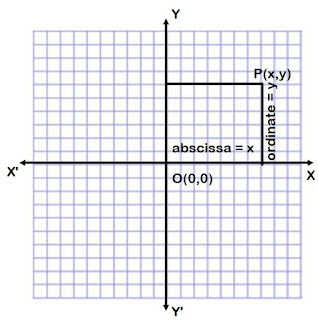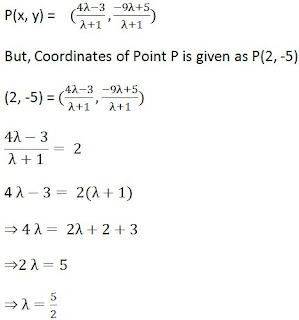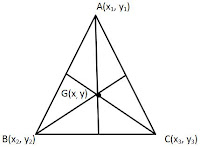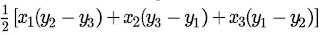## Revision Notes for Ch 7 Coordinate Geometry Class 10th Mathematics

Coordinate Geometry

• Coordinate Geometry: The branch of Mathematics in which algebraic methods are used to solve geometrical problems is known as coordinate geometry.

Coordinate System

• As shown in the figure, the line XOX′ is known as the X-axis and YOY′ is known as Y-axis.

• The point O is called the origin. For any point P (x y), the ordered pair (x y) is called the coordinate of point P.

• The distance of a point from Y-axis is called its abscissa and the distance of a point from X-axis is called its ordinate.• Distance between Two Points
The distance between two points P (x1, y1) and Q (x2, y2) is given by:This is also known as Distance Formula.
Note: The distance of any point P(x,y) from the origin O(0,0) is given by:• Section Formula

Let P (x,y) be a point on the line segment joining A(x1, y1) and B(x2, y2) such that it divides AB internally in the ratio m:n. The coordinates of the point P are given byThis is known as Section Formula.

Note:
(i) If the point P divides the line segment joining A(x1, y1) and B(x2, y2) internally in the ratio k:1, its coordinates are given by:Example: In what ratio does the point (2,- 5) divide the line segment joining the points A(-3, 5) and B(4, -9).

Solution:

Let the ratio be λ : 1The required ratio is 5:2.

Mid -Point Formula

The mid-point of the line joining A(x1, y1) and B(x2, y2) is given asCentroid of the Triangle
The Centroid of the triangle is the point of intersection of medians of the triangle. For a triangle A(x1, y1), B(x2, y2) and C(x3, y3) we can say that the co-ordinates of centroid is given asAreas of TriangleCondition for Collinearity of three Points